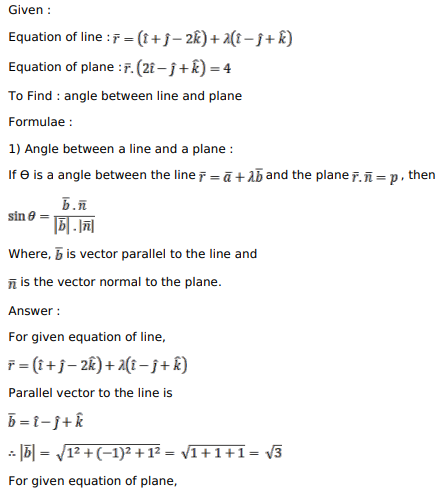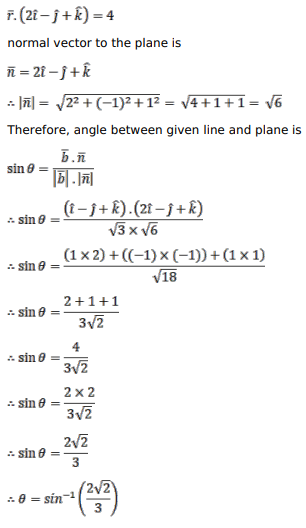# Find the angle between the line

Question:

Find the angle between the line $\bar{r}-(\hat{i}+\hat{j}-2 \hat{k})+\lambda(\hat{i}-\hat{j}+\hat{k})$ and the plane $\bar{r} \cdot(2 \hat{i}-\hat{j}+\hat{k})=4$.

Solution: